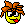# Combination maths problem

#### Irvin

##### MemberHello all,

I have the following question;

How many combinations are there when you have the following number limits per postion;

pos1= 1 to 13
pos 2= 3 to 22
pos 3= 7 to 29
pos 4= 12 to 35
pos 5= 19 to 38
pos 6= 27 to 40

I seem to be going crazy or brain dead at the moment.

Any help would be appreciated.

Please show all working so I can add to my spreadsheet.Thank you.

#### NmbrsDude

##### Member
I'm no mathematician but try this on for size...

By using the ranges you gave we obtain that each position can only have the following possible number of outcomes:

P1 13
P2 20
P3 23
P4 24
P5 20
P6 14

Therefore, the odds should be

13 x 20 x 23 x 24 x 20 x 14
6 x 5 x 4 x 3 x 2 x 1

which give us

40185600
720

which gives us odds of 1 in 55,813.3.

Hope this helps,
ND#### savagegoose

##### Member
yup the way to look at it is for the 1st posistion there are 6 possible results that you will win from. as the draw doesnt need to be ordered.

so thats 6 out of 13 or 13/6

for second pos there are only 5 balls remaining to be drawn so

its x/5

etc.

#### GillesD

##### Member
Possible combinations

Based on the requirements you specified, I calculated all possible combinations meeting those requirements.

I found 2,752,024 combinations from 1, 3, 7, 12, 19 and 27 to 13, 22, 29, 35, 38 and 40. This represents 71.7% of the 3,838,380 possible combinations of a 6/40 lottery.

But this time I am not that 100% sure of my program. I will run it to verify if these calculations are right. In all cases, I assumed that the number in second position is greater than the one in first, that the number in third position is greater than the one in second, and so on.

Last edited:

#### Irvin

##### Member
Thanks for the answers guys.

I originally thought it was around 55000 but have to go with GillesD answer.

If we anchor 1 to the first spot we end up with around 370,000 combinations. And percentage wise this corresponds with the numbers / position drawn to date.

Thanks again.